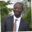Related Tags

javascript
compare
communitycreator

# How to use soft equality versus hard equality in JavaScriptAbel Lifaefi Mbula

### Overview

We often need to compare values when we are programming. There is more than one way to check for equality in JavaScript. We can use a double equals operator (==), also known as soft equality. We can also use the triple equals operator (===), also known as hard equality.

Let’s look at them in more detail.

### Soft equality

Soft equality only checks for equality in value, not in type.

For example, we can assign the number three to a variable like this: const a = 3;

We can compare it like so:

> a == 3
true


This works fine, but there are some slight problems with the double equals operator.

Let’s test again with the string "3":

> a == "3"
true


### Explanation

As you can see here, the returned output is true when in reality it should be false because a is not a string but a number.

So what happened that we got true?

When using soft equality, JavaScript will attempt to coerce the two values to the same type when making the comparison. This behavior of JavaScript can lead to some very strange results.

Let’s look at some more examples.

" " == 0;
<< true

" " == "0";
<< false

false == "0";
<< true

"1" == true;
<< true

"2" == true;
<< false

"true" == true;
<< false

null == undefined;
<< true


The snippet above shows values that are not equal, yet tend to be reported as being equal.

### Hard equality

Unlike soft equality, hard equality checks for both the value and the type. It is also called a stricter equality test and returns true only if the two elements are the same.

Let’s use the same example as above with hard equality and see how it will behave.

a === 3;
<< true

a === "3";
<< false

null === undefined
<< false


As you can see, a is equal to the number 3, but not equal to the string 3. The hard equality operator also correctly reports that null and undefined are two different values.

### Which one to use?

This is a good question, and the simple and quick answer is that it depends upon your need.

If your program does not need to check for the value type before doing operations (i.e., you’re certain that both sides will still have the same type), you can go for soft equality.

In the other case and by default, I would suggest always go for hard equality. Using soft equality can cause bugs in the program, and it is often difficult to discover them.

RELATED TAGS

javascript
compare
communitycreator

CONTRIBUTORAbel Lifaefi Mbula
RELATED COURSES

View all Courses

Keep Exploring

Learn in-demand tech skills in half the time